# collisionVHACD

Decompose mesh into convex collision meshes using V-HACD

Since R2023b

## Syntax

``convexCollMeshes = collisionVHACD(sourceMesh)``
``convexCollMeshes = collisionVHACD(sourceMesh,options)``
``[convexCollMeshes,info] = collisionVHACD(___)``

## Description

example

````convexCollMeshes = collisionVHACD(sourceMesh)` decomposes a triangulation mesh into convex collision meshes using a voxelized hierarchical approximate convex decomposition (V-HACD) solver.```

example

````convexCollMeshes = collisionVHACD(sourceMesh,options)` specifies the solver options of the V-HACD solver.```
````[convexCollMeshes,info] = collisionVHACD(___)` returns information about the decomposition. in addition to the decomposed convex collision meshes, using any combination of input arguments from previous syntaxes.```

## Examples

collapse all

Load an STL file containing a rectangular bin triangulation, and then visualize the bin triangulation.

```meshTri = stlread("bin.stl"); trisurf(meshTri) axis equal```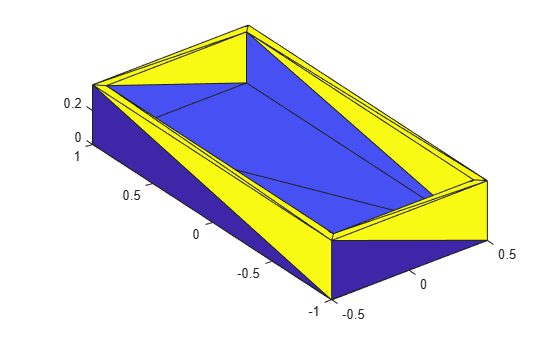Create a collision mesh using the points from the triangulation of the bin, and then visualize the mesh. Note that, when you approximate the bin triangulation as one collision mesh, the `collisionMesh` object uses the convex hull of the bin triangulation to approximate the bin. As a result the collision mesh is convex, unlike the non-convex bin triangulation. The `collisionMesh` object does this because collision checking is most efficient with convex meshes. However, this convex approximation is not ideal for bins because robots can manipulate bins or objects inside of bins.

```meshColl = collisionMesh(meshTri.Points); [~,p] = show(meshColl); view(145,30) % Change view so it is easier to view the inside of bin axis equal hold on```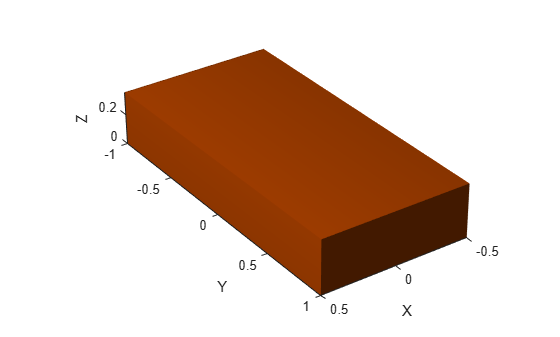Create a soda can using a collision cylinder, and set the pose such that it sits in the center of the bin. Then, show it in the convex collision mesh.

```sodacan = collisionCylinder(0.1,0.4,Pose=trvec2tform([0 0 .3])); show(sodacan);```

Set the box to be transparent so that you can see the overlap between the bin and the soda can.

```p.FaceAlpha = 0.25; hold off```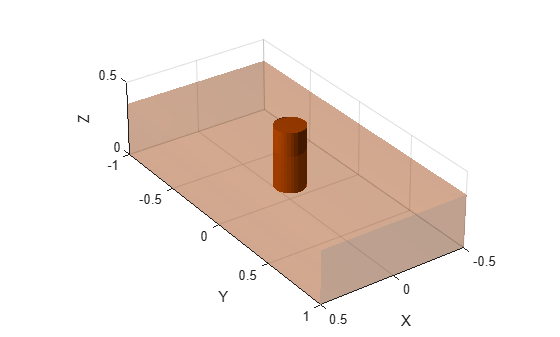Check collision between the soda can and the convex approximation of the bin, and note that they are in collision.

`isCollidingConvex = checkCollision(sodacan,meshColl)`
```isCollidingConvex = 1 ```

To get a better approximation of the bin for collision checking, decompose the original non-convex mesh into multiple convex meshes using voxelized hierarchical approximate convex decomposition (V-HACD).

Use the `collisionVHACD` function to decompose the original non-convex triangulation into convex collision meshes. Then, show the decomposed bin with the soda can.

```decomposedBin = collisionVHACD(meshTri); showCollisionArray([decomposedBin {sodacan}]); view(145,30) axis equal```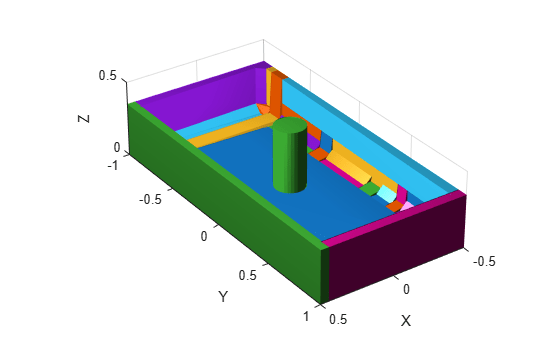Check collision with all the meshes that approximate the bin. Note that the soda can is not in collision with the decomposed non-convex approximation of the bin. If you require a more accurate decomposition of the bin, you can specify custom solver options using the `vhacdOptions` object.

```isColliding = false(1,length(decomposedBin)); for i = 1:length(decomposedBin) isColliding(i) = checkCollision(sodacan,decomposedBin{i}); end isCollidingAll = all(isColliding)```
```isCollidingAll = logical 0 ```

Load the Rethink Robotics Sawyer robot.

`robot = loadrobot("rethinkSawyer",DataFormat="row")`
```robot = rigidBodyTree with properties: NumBodies: 20 Bodies: {1x20 cell} Base: [1x1 rigidBody] BodyNames: {1x20 cell} BaseName: 'base' Gravity: [0 0 0] DataFormat: 'row' ```

Show the robot with just the visual meshes and show the robot with just the collision meshes. Use a vertical view so the difference between the arms is more clear.

```tiledlayout(1,2) sgtitle("Rethink Robotics Sawyer") nexttile show(robot,Visuals="on",Collisions="off"); title("Top Down View") axis auto view(90,90) nexttile show(robot,Visuals="off",Collisions="on"); title("Top Down View") axis auto view(90,90)```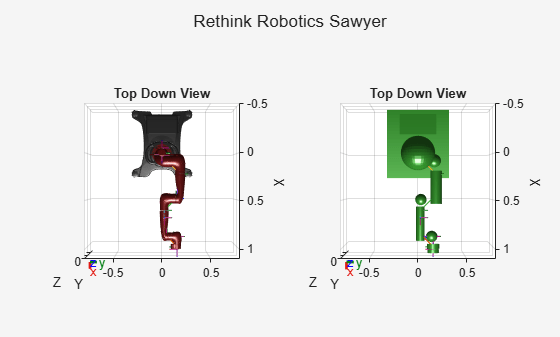Note that each body of the arm is represented by a single convex mesh that does not accurately represent the physical boundaries of the arm. To achieve more accurate collision checking, you must decompose the visual meshes of the robot. The rigid body tree stores the rigid bodies of the arm at indices `9` to `17`.

First, create V-HACD solver options for individual mesh decompositions with the maximum number of convex hulls set to `10`.

`opts = vhacdOptions("IndividualMesh",MaxNumConvexHulls=10);`

Then for each rigid body:

1. Get the current rigid body and clear the current collision mesh.

2. Get the corresponding visual data if there is any.

3. If there is visual data, use `collisionVHACD` with the custom solver options to decompose the triangulation of the visual data into an array of collision meshes.

4. Add each collision mesh from the array of collision meshes to the rigid body.

```for bodyIdxToReplace = 9:17 % 1. Get current body and clear collision mesh currBody = robot.Bodies{bodyIdxToReplace}; clearCollision(currBody); % 2. Get Corresponding visual data vizData = getVisual(robot.Bodies{bodyIdxToReplace}); % 3. If visual data, decompose visual data if ~isempty(vizData) collisionArray = collisionVHACD(vizData(1).Triangulation,opts); % 4. Add each collision mesh to the rigid body for j = 1:numel(collisionArray) addCollision(currBody,collisionArray{j}); end end end```

Show the original collision meshes of the robot arm next to the updated collision mesh of the arm.

```tiledlayout(1,2); sgtitle("Rethink Robotics Sawyer") nexttile robotOriginal = loadrobot("rethinkSawyer",DataFormat="row"); show(robotOriginal,Visuals="off",Collisions="on"); title("Before Decomposition") axis auto view(90,90) nexttile show(robot,Visuals="off",Collisions="on"); title("After Decomposition") view(90,90) axis auto```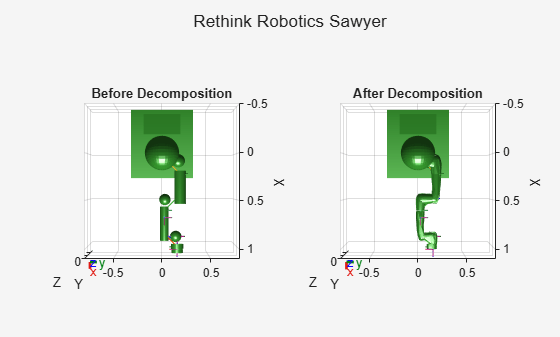Note that in this case the new collision meshes represent the robot arm more accurately.

## Input Arguments

collapse all

Triangulation of mesh geometry to decompose, specified as a `triangulation` object.

For visualizing triangulations of meshes before decomposition, you can use the `trimesh` or `trisurf` function.

V-HACD solver options, specified as a `vhacdOptions` object.

You must set the `Type` property of the `vhacdOptions` object to `"IndividualMesh"` to specify custom V-HACD options for the `collisionVHACD` function. If the `Type` property is set to `"RigidBodyTree"`, then you can only use the `vhacdOptions` object for importing rigid body trees using the `importrobot` function

## Output Arguments

collapse all

Convex collision meshes, returned as an N-element cell array of `collisionMesh` objects.

You can use the `showCollisionArray` function to visualize the collision meshes.

Decomposition solution information, returned as a structure containing these fields:

• `SourceVolume` — Volume of the input source mesh specified by the `sourceMesh` argument.

• `CompositeDecompVolume` — Total combined volume of the output convex collision meshes. This total includes the volume of overlapping meshes.

• `RawData`N-element cell array of mesh vertices used to generate the collision array. N is the total number of collision objects needed to represent the decomposed mesh.

 Mammou, Khaled, et al. “Voxelized Hierarchical Approximate Convex Decomposition - V-HACD Version 4.” GitHub, October 24, 2022. https://github.com/kmammou/v-hacd.